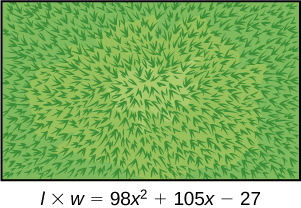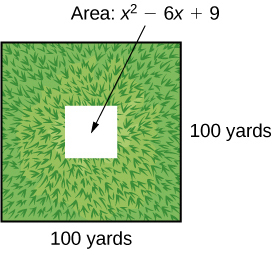# 1.5 Factoring polynomials  (Page 4/6)

 Page 4 / 6

Given a sum of cubes or difference of cubes, factor it.

1. Confirm that the first and last term are cubes, $\text{\hspace{0.17em}}{a}^{3}+{b}^{3}\text{\hspace{0.17em}}$ or $\text{\hspace{0.17em}}{a}^{3}-{b}^{3}.$
2. For a sum of cubes, write the factored form as $\text{\hspace{0.17em}}\left(a+b\right)\left({a}^{2}-ab+{b}^{2}\right).\text{\hspace{0.17em}}$ For a difference of cubes, write the factored form as $\text{\hspace{0.17em}}\left(a-b\right)\left({a}^{2}+ab+{b}^{2}\right).$

## Factoring a sum of cubes

Factor $\text{\hspace{0.17em}}{x}^{3}+512.$

Notice that $\text{\hspace{0.17em}}{x}^{3}\text{\hspace{0.17em}}$ and $\text{\hspace{0.17em}}512\text{\hspace{0.17em}}$ are cubes because $\text{\hspace{0.17em}}{8}^{3}=512.\text{\hspace{0.17em}}$ Rewrite the sum of cubes as $\text{\hspace{0.17em}}\left(x+8\right)\left({x}^{2}-8x+64\right).$

Factor the sum of cubes: $\text{\hspace{0.17em}}216{a}^{3}+{b}^{3}.$

$\left(6a+b\right)\left(36{a}^{2}-6ab+{b}^{2}\right)$

## Factoring a difference of cubes

Factor $\text{\hspace{0.17em}}8{x}^{3}-125.$

Notice that $\text{\hspace{0.17em}}8{x}^{3}\text{\hspace{0.17em}}$ and $\text{\hspace{0.17em}}125\text{\hspace{0.17em}}$ are cubes because $\text{\hspace{0.17em}}8{x}^{3}={\left(2x\right)}^{3}\text{\hspace{0.17em}}$ and $\text{\hspace{0.17em}}125={5}^{3}.\text{\hspace{0.17em}}$ Write the difference of cubes as $\text{\hspace{0.17em}}\left(2x-5\right)\left(4{x}^{2}+10x+25\right).$

Factor the difference of cubes: $\text{\hspace{0.17em}}1,000{x}^{3}-1.$

$\left(10x-1\right)\left(100{x}^{2}+10x+1\right)$

## Factoring expressions with fractional or negative exponents

Expressions with fractional or negative exponents can be factored by pulling out a GCF. Look for the variable or exponent that is common to each term of the expression and pull out that variable or exponent raised to the lowest power. These expressions follow the same factoring rules as those with integer exponents. For instance, $\text{\hspace{0.17em}}2{x}^{\frac{1}{4}}+5{x}^{\frac{3}{4}}\text{\hspace{0.17em}}$ can be factored by pulling out $\text{\hspace{0.17em}}{x}^{\frac{1}{4}}\text{\hspace{0.17em}}$ and being rewritten as $\text{\hspace{0.17em}}{x}^{\frac{1}{4}}\left(2+5{x}^{\frac{1}{2}}\right).$

## Factoring an expression with fractional or negative exponents

Factor $\text{\hspace{0.17em}}3x{\left(x+2\right)}^{\frac{-1}{3}}+4{\left(x+2\right)}^{\frac{2}{3}}.$

Factor out the term with the lowest value of the exponent. In this case, that would be $\text{\hspace{0.17em}}{\left(x+2\right)}^{-\frac{1}{3}}.$

Factor $\text{\hspace{0.17em}}2{\left(5a-1\right)}^{\frac{3}{4}}+7a{\left(5a-1\right)}^{-\frac{1}{4}}.$

${\left(5a-1\right)}^{-\frac{1}{4}}\left(17a-2\right)$

Access these online resources for additional instruction and practice with factoring polynomials.

## Key equations

 difference of squares ${a}^{2}-{b}^{2}=\left(a+b\right)\left(a-b\right)$ perfect square trinomial ${a}^{2}+2ab+{b}^{2}={\left(a+b\right)}^{2}$ sum of cubes ${a}^{3}+{b}^{3}=\left(a+b\right)\left({a}^{2}-ab+{b}^{2}\right)$ difference of cubes ${a}^{3}-{b}^{3}=\left(a-b\right)\left({a}^{2}+ab+{b}^{2}\right)$
• The greatest common factor, or GCF, can be factored out of a polynomial. Checking for a GCF should be the first step in any factoring problem. See [link] .
• Trinomials with leading coefficient 1 can be factored by finding numbers that have a product of the third term and a sum of the second term. See [link] .
• Trinomials can be factored using a process called factoring by grouping. See [link] .
• Perfect square trinomials and the difference of squares are special products and can be factored using equations. See [link] and [link] .
• The sum of cubes and the difference of cubes can be factored using equations. See [link] and [link] .
• Polynomials containing fractional and negative exponents can be factored by pulling out a GCF. See [link] .

## Verbal

If the terms of a polynomial do not have a GCF, does that mean it is not factorable? Explain.

The terms of a polynomial do not have to have a common factor for the entire polynomial to be factorable. For example, $\text{\hspace{0.17em}}4{x}^{2}\text{\hspace{0.17em}}$ and $\text{\hspace{0.17em}}-9{y}^{2}\text{\hspace{0.17em}}$ don’t have a common factor, but the whole polynomial is still factorable: $\text{\hspace{0.17em}}4{x}^{2}-9{y}^{2}=\left(2x+3y\right)\left(2x-3y\right).$

A polynomial is factorable, but it is not a perfect square trinomial or a difference of two squares. Can you factor the polynomial without finding the GCF?

How do you factor by grouping?

Divide the $\text{\hspace{0.17em}}x\text{\hspace{0.17em}}$ term into the sum of two terms, factor each portion of the expression separately, and then factor out the GCF of the entire expression.

## Algebraic

For the following exercises, find the greatest common factor.

$14x+4xy-18x{y}^{2}$

$49m{b}^{2}-35{m}^{2}ba+77m{a}^{2}$

$7m$

$30{x}^{3}y-45{x}^{2}{y}^{2}+135x{y}^{3}$

$200{p}^{3}{m}^{3}-30{p}^{2}{m}^{3}+40{m}^{3}$

$10{m}^{3}$

$36{j}^{4}{k}^{2}-18{j}^{3}{k}^{3}+54{j}^{2}{k}^{4}$

$6{y}^{4}-2{y}^{3}+3{y}^{2}-y$

$y$

For the following exercises, factor by grouping.

$6{x}^{2}+5x-4$

$2{a}^{2}+9a-18$

$\left(2a-3\right)\left(a+6\right)$

$6{c}^{2}+41c+63$

$6{n}^{2}-19n-11$

$\left(3n-11\right)\left(2n+1\right)$

$20{w}^{2}-47w+24$

$2{p}^{2}-5p-7$

$\left(p+1\right)\left(2p-7\right)$

For the following exercises, factor the polynomial.

$7{x}^{2}+48x-7$

$10{h}^{2}-9h-9$

$\left(5h+3\right)\left(2h-3\right)$

$2{b}^{2}-25b-247$

$9{d}^{2}-73d+8$

$\left(9d-1\right)\left(d-8\right)$

$90{v}^{2}-181v+90$

$12{t}^{2}+t-13$

$\left(12t+13\right)\left(t-1\right)$

$2{n}^{2}-n-15$

$16{x}^{2}-100$

$\left(4x+10\right)\left(4x-10\right)$

$25{y}^{2}-196$

$121{p}^{2}-169$

$\left(11p+13\right)\left(11p-13\right)$

$4{m}^{2}-9$

$361{d}^{2}-81$

$\left(19d+9\right)\left(19d-9\right)$

$324{x}^{2}-121$

$144{b}^{2}-25{c}^{2}$

$\left(12b+5c\right)\left(12b-5c\right)$

$16{a}^{2}-8a+1$

$49{n}^{2}+168n+144$

${\left(7n+12\right)}^{2}$

$121{x}^{2}-88x+16$

$225{y}^{2}+120y+16$

${\left(15y+4\right)}^{2}$

${m}^{2}-20m+100$

${m}^{2}-20m+100$

${\left(5p-12\right)}^{2}$

$36{q}^{2}+60q+25$

For the following exercises, factor the polynomials.

${x}^{3}+216$

$\left(x+6\right)\left({x}^{2}-6x+36\right)$

$27{y}^{3}-8$

$125{a}^{3}+343$

$\left(5a+7\right)\left(25{a}^{2}-35a+49\right)$

${b}^{3}-8{d}^{3}$

$64{x}^{3}-125$

$\left(4x-5\right)\left(16{x}^{2}+20x+25\right)$

$729{q}^{3}+1331$

$125{r}^{3}+1,728{s}^{3}$

$\left(5r+12s\right)\left(25{r}^{2}-60rs+144{s}^{2}\right)$

$4x{\left(x-1\right)}^{-\frac{2}{3}}+3{\left(x-1\right)}^{\frac{1}{3}}$

$3c{\left(2c+3\right)}^{-\frac{1}{4}}-5{\left(2c+3\right)}^{\frac{3}{4}}$

${\left(2c+3\right)}^{-\frac{1}{4}}\left(-7c-15\right)$

$3t{\left(10t+3\right)}^{\frac{1}{3}}+7{\left(10t+3\right)}^{\frac{4}{3}}$

$14x{\left(x+2\right)}^{-\frac{2}{5}}+5{\left(x+2\right)}^{\frac{3}{5}}$

${\left(x+2\right)}^{-\frac{2}{5}}\left(19x+10\right)$

$9y{\left(3y-13\right)}^{\frac{1}{5}}-2{\left(3y-13\right)}^{\frac{6}{5}}$

$5z{\left(2z-9\right)}^{-\frac{3}{2}}+11{\left(2z-9\right)}^{-\frac{1}{2}}$

${\left(2z-9\right)}^{-\frac{3}{2}}\left(27z-99\right)$

$6d{\left(2d+3\right)}^{-\frac{1}{6}}+5{\left(2d+3\right)}^{\frac{5}{6}}$

## Real-world applications

For the following exercises, consider this scenario:

Charlotte has appointed a chairperson to lead a city beautification project. The first act is to install statues and fountains in one of the city’s parks. The park is a rectangle with an area of $\text{\hspace{0.17em}}98{x}^{2}+105x-27\text{\hspace{0.17em}}$ m 2 , as shown in the figure below. The length and width of the park are perfect factors of the area.Factor by grouping to find the length and width of the park.

$\left(14x-3\right)\left(7x+9\right)$

A statue is to be placed in the center of the park. The area of the base of the statue is $\text{\hspace{0.17em}}4{x}^{2}+12x+9{\text{m}}^{2}.\text{\hspace{0.17em}}$ Factor the area to find the lengths of the sides of the statue.

At the northwest corner of the park, the city is going to install a fountain. The area of the base of the fountain is $\text{\hspace{0.17em}}9{x}^{2}-25{\text{m}}^{2}.\text{\hspace{0.17em}}$ Factor the area to find the lengths of the sides of the fountain.

$\left(3x+5\right)\left(3x-5\right)$

For the following exercise, consider the following scenario:

A school is installing a flagpole in the central plaza. The plaza is a square with side length 100 yd. as shown in the figure below. The flagpole will take up a square plot with area $\text{\hspace{0.17em}}{x}^{2}-6x+9$ yd 2 .Find the length of the base of the flagpole by factoring.

## Extensions

For the following exercises, factor the polynomials completely.

$16{x}^{4}-200{x}^{2}+625$

${\left(2x+5\right)}^{2}{\left(2x-5\right)}^{2}$

$81{y}^{4}-256$

$16{z}^{4}-2,401{a}^{4}$

$\left(4{z}^{2}+49{a}^{2}\right)\left(2z+7a\right)\left(2z-7a\right)$

$5x{\left(3x+2\right)}^{-\frac{2}{4}}+{\left(12x+8\right)}^{\frac{3}{2}}$

${\left(32{x}^{3}+48{x}^{2}-162x-243\right)}^{-1}$

$\frac{1}{\left(4x+9\right)\left(4x-9\right)\left(2x+3\right)}$

root under 3-root under 2 by 5 y square
The sum of the first n terms of a certain series is 2^n-1, Show that , this series is Geometric and Find the formula of the n^th
cosA\1+sinA=secA-tanA
why two x + seven is equal to nineteen.
The numbers cannot be combined with the x
Othman
2x + 7 =19
humberto
2x +7=19. 2x=19 - 7 2x=12 x=6
Yvonne
because x is 6
SAIDI
what is the best practice that will address the issue on this topic? anyone who can help me. i'm working on my action research.
simplify each radical by removing as many factors as possible (a) √75
how is infinity bidder from undefined?
what is the value of x in 4x-2+3
give the complete question
Shanky
4x=3-2 4x=1 x=1+4 x=5 5x
Olaiya
hi can you give another equation I'd like to solve it
Daniel
what is the value of x in 4x-2+3
Olaiya
if 4x-2+3 = 0 then 4x = 2-3 4x = -1 x = -(1÷4) is the answer.
Jacob
4x-2+3 4x=-3+2 4×=-1 4×/4=-1/4
LUTHO
then x=-1/4
LUTHO
4x-2+3 4x=-3+2 4x=-1 4x÷4=-1÷4 x=-1÷4
LUTHO
A research student is working with a culture of bacteria that doubles in size every twenty minutes. The initial population count was  1350  bacteria. Rounding to five significant digits, write an exponential equation representing this situation. To the nearest whole number, what is the population size after  3  hours?
v=lbh calculate the volume if i.l=5cm, b=2cm ,h=3cm
Need help with math
Peya
can you help me on this topic of Geometry if l help you
litshani
( cosec Q _ cot Q ) whole spuare = 1_cosQ / 1+cosQ
A guy wire for a suspension bridge runs from the ground diagonally to the top of the closest pylon to make a triangle. We can use the Pythagorean Theorem to find the length of guy wire needed. The square of the distance between the wire on the ground and the pylon on the ground is 90,000 feet. The square of the height of the pylon is 160,000 feet. So, the length of the guy wire can be found by evaluating √(90000+160000). What is the length of the guy wire?
the indicated sum of a sequence is known as
how do I attempted a trig number as a starter
cos 18 ____ sin 72 evaluateByByBy David CoreyBy Edgar DelgadoBy Madison ChristianBy Savannah ParrishBy Tamsin KnoxBy OpenStaxBy Stephen VoronBy Rylee MinllicBy Jonathan LongBy Rohini Ajay